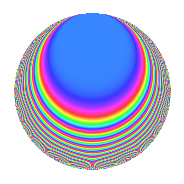# Properties

 Label 201.1.k.aLevel 201 Weight 1 Character orbit 201.k Analytic conductor 0.100 Analytic rank 0 Dimension 10 Projective image $$D_{11}$$ CM disc. -3 Inner twists 4

# Related objects

## Newspace parameters

 Level: $$N$$ = $$201 = 3 \cdot 67$$ Weight: $$k$$ = $$1$$ Character orbit: $$[\chi]$$ = 201.k (of order $$22$$ and degree $$10$$)

## Newform invariants

 Self dual: No Analytic conductor: $$0.100312067539$$ Analytic rank: $$0$$ Dimension: $$10$$ Coefficient field: $$\Q(\zeta_{22})$$ Coefficient ring: $$\Z[a_1, a_2, a_3]$$ Coefficient ring index: $$1$$ Projective image $$D_{11}$$ Projective field Galois closure of $$\mathbb{Q}[x]/(x^{11} - \cdots)$$

## $q$-expansion

The $$q$$-expansion and trace form are shown below.

 $$f(q)$$ $$=$$ $$q -\zeta_{22}^{7} q^{3} + \zeta_{22}^{6} q^{4} + ( \zeta_{22}^{8} - \zeta_{22}^{9} ) q^{7} -\zeta_{22}^{3} q^{9} +O(q^{10})$$ $$q -\zeta_{22}^{7} q^{3} + \zeta_{22}^{6} q^{4} + ( \zeta_{22}^{8} - \zeta_{22}^{9} ) q^{7} -\zeta_{22}^{3} q^{9} + \zeta_{22}^{2} q^{12} + ( -\zeta_{22}^{5} + \zeta_{22}^{10} ) q^{13} -\zeta_{22} q^{16} + ( -\zeta_{22} + \zeta_{22}^{4} ) q^{19} + ( \zeta_{22}^{4} - \zeta_{22}^{5} ) q^{21} + \zeta_{22}^{2} q^{25} + \zeta_{22}^{10} q^{27} + ( -\zeta_{22}^{3} + \zeta_{22}^{4} ) q^{28} + ( \zeta_{22}^{8} + \zeta_{22}^{10} ) q^{31} -\zeta_{22}^{9} q^{36} + ( \zeta_{22}^{2} - \zeta_{22}^{9} ) q^{37} + ( -\zeta_{22} + \zeta_{22}^{6} ) q^{39} + ( -\zeta_{22}^{3} - \zeta_{22}^{7} ) q^{43} + \zeta_{22}^{8} q^{48} + ( -\zeta_{22}^{5} + \zeta_{22}^{6} - \zeta_{22}^{7} ) q^{49} + ( 1 - \zeta_{22}^{5} ) q^{52} + ( 1 + \zeta_{22}^{8} ) q^{57} + ( -\zeta_{22}^{3} + \zeta_{22}^{6} ) q^{61} + ( 1 - \zeta_{22} ) q^{63} -\zeta_{22}^{7} q^{64} + \zeta_{22}^{2} q^{67} + ( 1 - \zeta_{22}^{9} ) q^{73} -\zeta_{22}^{9} q^{75} + ( -\zeta_{22}^{7} + \zeta_{22}^{10} ) q^{76} + ( 1 + \zeta_{22}^{4} ) q^{79} + \zeta_{22}^{6} q^{81} + ( 1 + \zeta_{22}^{10} ) q^{84} + ( \zeta_{22}^{2} - \zeta_{22}^{3} - \zeta_{22}^{7} + \zeta_{22}^{8} ) q^{91} + ( \zeta_{22}^{4} + \zeta_{22}^{6} ) q^{93} + ( \zeta_{22}^{4} - \zeta_{22}^{7} ) q^{97} +O(q^{100})$$ $$\operatorname{Tr}(f)(q)$$ $$=$$ $$10q - q^{3} - q^{4} - 2q^{7} - q^{9} + O(q^{10})$$ $$10q - q^{3} - q^{4} - 2q^{7} - q^{9} - q^{12} - 2q^{13} - q^{16} - 2q^{19} - 2q^{21} - q^{25} - q^{27} - 2q^{28} - 2q^{31} - q^{36} - 2q^{37} - 2q^{39} - 2q^{43} - q^{48} - 3q^{49} + 9q^{52} + 9q^{57} - 2q^{61} + 9q^{63} - q^{64} - q^{67} + 9q^{73} - q^{75} - 2q^{76} + 9q^{79} - q^{81} + 9q^{84} - 4q^{91} - 2q^{93} - 2q^{97} + O(q^{100})$$

## Character Values

We give the values of $$\chi$$ on generators for $$\left(\mathbb{Z}/201\mathbb{Z}\right)^\times$$.

 $$n$$ $$68$$ $$136$$ $$\chi(n)$$ $$-1$$ $$\zeta_{22}^{6}$$

## Embeddings

For each embedding $$\iota_m$$ of the coefficient field, the values $$\iota_m(a_n)$$ are shown below.

For more information on an embedded modular form you can click on its label.

Label $$\iota_m(\nu)$$ $$a_{2}$$ $$a_{3}$$ $$a_{4}$$ $$a_{5}$$ $$a_{6}$$ $$a_{7}$$ $$a_{8}$$ $$a_{9}$$ $$a_{10}$$
14.1
 0.142315 + 0.989821i −0.841254 − 0.540641i 0.959493 − 0.281733i −0.415415 + 0.909632i −0.841254 + 0.540641i 0.959493 + 0.281733i −0.415415 − 0.909632i 0.654861 − 0.755750i 0.654861 + 0.755750i 0.142315 − 0.989821i
0 0.841254 + 0.540641i −0.654861 + 0.755750i 0 0 −0.544078 1.19136i 0 0.415415 + 0.909632i 0
59.1 0 −0.654861 0.755750i −0.959493 0.281733i 0 0 0.273100 1.89945i 0 −0.142315 + 0.989821i 0
62.1 0 0.415415 + 0.909632i −0.142315 0.989821i 0 0 0.186393 0.215109i 0 −0.654861 + 0.755750i 0
89.1 0 −0.142315 0.989821i 0.841254 0.540641i 0 0 −1.61435 + 0.474017i 0 −0.959493 + 0.281733i 0
92.1 0 −0.654861 + 0.755750i −0.959493 + 0.281733i 0 0 0.273100 + 1.89945i 0 −0.142315 0.989821i 0
107.1 0 0.415415 0.909632i −0.142315 + 0.989821i 0 0 0.186393 + 0.215109i 0 −0.654861 0.755750i 0
131.1 0 −0.142315 + 0.989821i 0.841254 + 0.540641i 0 0 −1.61435 0.474017i 0 −0.959493 0.281733i 0
143.1 0 −0.959493 0.281733i 0.415415 + 0.909632i 0 0 0.698939 + 0.449181i 0 0.841254 + 0.540641i 0
149.1 0 −0.959493 + 0.281733i 0.415415 0.909632i 0 0 0.698939 0.449181i 0 0.841254 0.540641i 0
158.1 0 0.841254 0.540641i −0.654861 0.755750i 0 0 −0.544078 + 1.19136i 0 0.415415 0.909632i 0
 $$n$$: e.g. 2-40 or 990-1000 Embeddings: e.g. 1-3 or 158.1 Significant digits: Format: Complex embeddings Normalized embeddings Satake parameters Satake angles

## Inner twists

Char. orbit Parity Mult. Self Twist Proved
1.a Even 1 trivial yes
3.b Odd 1 CM by $$\Q(\sqrt{-3})$$ yes
67.e Even 1 yes
201.k Odd 1 yes

## Hecke kernels

There are no other newforms in $$S_{1}^{\mathrm{new}}(201, [\chi])$$.